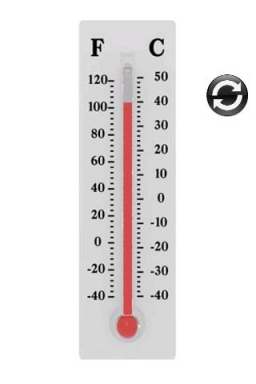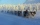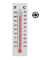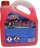# The temperature 2

The temperature of Siberia fell13 degrees below 2/3 degrees centigrad. What is the temperature there now?

t =  -12.3333 °C

### Step-by-step explanation:Did you find an error or inaccuracy? Feel free to write us. Thank you!## Related math problems and questions:

• The temperature 3The temperature is -12 degrees Fahrenheit. During the following 5 hours, the temperature decrease by 23 degrees Fahrenheit. What is the weather at 11 pm?
• The temperatureThe temperature is 0 degrees. It drops 5 degrees after 1 hour and then drops 3 degrees more the next hour. What is the temperature after 6 hours?
• Change in temperatureStarting temperature is 21°C, the highest temperature is 32°C. What is the change in temperature?
• Temperature 2Sunday's high temperature was 3 degrees higher than Saturday's. On Monday, the temperature fell 5 degrees, then rose 7 degrees on Tuesday and 4 more on Wednesday. Then it fell 17 degrees to a record low of 31 on Thursday. What was the temperature on Satur
• Degrees 2The temperature was 3°F and fell four degrees Fahrenheit. What is actually temperature?
• The temperature 8The temperature on Monday was –6°C. On Tuesday, the temperature was 3 degrees lower. Write down the temperature on Tuesday.
• Temperature change 3At 2 PM, The temperature was 76 degrees Fahrenheit. At 8 PM, the temperature was 58 degrees Fahrenheit. What was the change in temperature?
• Temperature up and dowmAt 6 AM, the temperature is 19.4°C. Between 6 AM and noon, the temperature rises 3.8°C. Between noon and 6 PM, the temperature falls 2.5°C. What is the temperature at 6 PM?
• Temperature changeThe mean temperature change is -3.2°F per day for five days. What is the total change over the five day period?
• Now isNow is 5°C morning. What will the temperature be if i. It rises by 12°C ii. It falls by 15°C
• The temperature 9The temperature at 9 AM is 2 degrees. The temperature rises 3 more degrees by noon. Which expression describes the temperature at noon? Calculate the new temperature value.
• The temperature 11The temperature on Sunday was 4°C. The temperature dropped 8°C on Monday and twice as much on Tuesday. What is the temperature on Tuesday?
• Outdoor temperatureThe outdoor temperature was 8 degrees at midnight. The temperature declined 5 degrees during each of the next 3 hours. What was the temperature at 3am?
• Celsius 25 degrees Celsius at midday dropped 12°Celsius degree by evening. What is the temperature?
• Temperature variationsToday's temperature was 80 degrees, and then the temperature dropped 10 degrees. Then it dropped 15 degrees again, then the next day, the temperature went up 2 degrees. What would the temperature be?
• Temperature rise and fallOn Friday, the temperature was 82°F. The temperature changed by –2°F on Saturday, and then it changed by 5°F on Sunday. What was the temperature on Sunday? How did the temperature change? Note we consider the mathematical problem formulated in this way to
• The temperature 10The temperature in a freezer is -15°C, and it increases by 3°C.